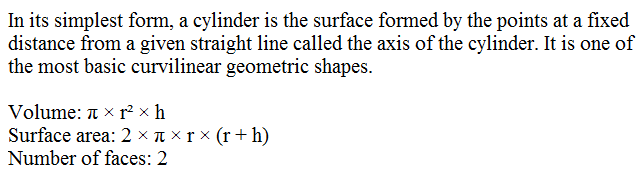# Java Program Volume Of Cylinder | 3 simple ways

Java program to find the volume of a cylinder with examples. Here, we shared three to four basic programs about to calculate the volume of a cylinder. If you have any doubts related to this programming then do comment at the end of the post we are glad to help you out – check java program to find the area of a triangle

1. Example # 1 – A simple java program universally applicable.
2. Example # 2 – Using a command line argument # universally applicable.
3. Example # 3 – Using a static method – # Universally applicable.

# check out the online compiler and execution tool for each example program #

What is a cylinder?

A geometrical figure with straight parallel sides and a circular cross-section. For detailed information check out the below definition.What is the formula to find out the volume of a cylinder?

A : The basic formula in order to calculate the volume of cylinder as follows :

Volume: π × r² × h

Here is the sample program # 1 : # The following java program is tested and executed in cmd. You can also check execute in the below compiler tool. #calculate volume of prism java code #

Output :

Online execution tool for the above program #example -1

Here is the complete explanation of above java  code. If you at the beginning stage, then you can check out the below tutorial. so that you will get an idea. If you know how the code works , then you can check out the rest of the examples with online compiler and execution tool.

– System read the input variables and pass the necessary methods to a new scanner.

– It is a blueprint where objects are created within a class.

– The main function , convert the given string as an argument into different formats as like double . int , float.

– Read the input values, where system.in can read the values from your system(import java.util.scanner) JDK file.

– Display the output values , in that whatever you were written.

– Where double is a datatype , which can store the particular values.

– The formula to find out the volume of a cylinder in java.

– Output displayed here.

So, you got an idea how the above java program works to find the volume of a cylinder. Below is another example method to calculate the volume of a cylinder. The difference between the above example -1 when compared with the below example -2 is nothing but just a couple of syntax lines has been changed. The little difference is as, whereas scanner function is used in order to retrieve the input variables and store in a particular location until the program executes. Whereas here, ‘parse’ method is used to directly retrieve the details from the screen itself.

Compiler method in # CMD: java filename.java

Execution method in #CMD: java classname arguments

Here is the Example program # 2 – using command line argument

Here is the online execution tool for the above program to calculate the volume of a cylinder.

Here is another example method #3 to calculate the volume. In the following method, we used a return invoked method. Check it out.

Example method # 3

# java program to calculate the volume of cylinder ends #

x

## X Star Pattern Java Program – Patterns

Java program to print X star pattern program – We have written the below print/draw ...